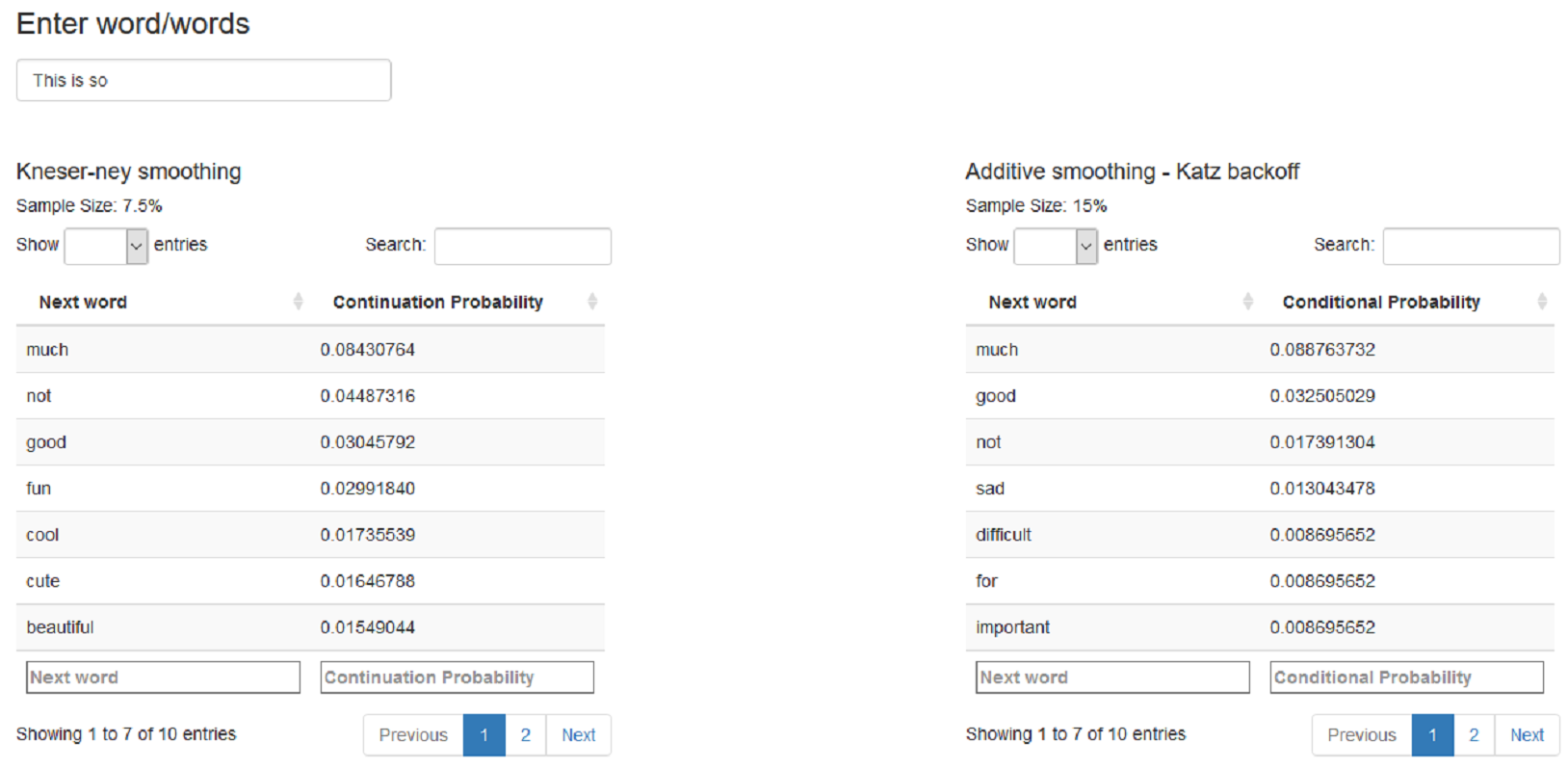# PredictNextWord

Tinniam V Ganesh
22 Aug 2015

### Create and clean the Corpus

This presentation highlights the steps in creating a Word Predict Shiny App

• Ingest the from the Tweets, Blogs and News
• Sample the data train & test data (7.5% for Kneser-Ney smoothing & 10% for Additiive smoothing-Katz backoff)
• Create a Corpus from the tweets, blogs and news items
• Clean the Corpus to remove punctuation, special characters, stopwords etc
• Remove profanity from the training and test set
• Use the package RWeka to create Quadgrams,Trigrams,Bigrams and Unigrams

### Use Laplace Add-1 smoothing & Katz backoff

1. Use Markov chains to calculate the Maximum Likelihood estimate P(C|AB) = count(ABC)/count(AB)
2. For previous terms whose count is 0, perform Laplace Add - 1 smoothing Padd-1(C|AB) = (count(C|AB) + 1)/(count(AB) + V)
3. Use Katz backoff algorithm to back off to lower n-1 grams if not found in n grams
4. Create n-gram csv files with n-1 gram, next word and conditional probability

### Kneser-Ney smoothing

The Kneser-Ney smoothing is based on determining the 'continuation probability' of the next word.

The Kneser-Ney formula is given below $$P_{\mathit{KN}}(w_i \mid w_{i-1}) = \dfrac{\max(c(w_{i-1} w_i) - \delta, 0)}{\sum_{w'} c(w_{i-1} w')} + \lambda \dfrac{\left| \{ w_{i-1} : c(w_{i-1}, w_i) > 0 \} \right|}{\left| \{ w_{j-1} : c(w_{j-1},w_j) > 0\} \right|}$$ where $$\delta$$ is the 'discount' and $$\lambda$$ is a normalizing constant

$$\lambda(w_{i-1}) = \dfrac{\delta}{c(w_{i-1})} \left| \{w' : c(w_{i-1}, w') > 0\} \right|.$$

Create n-grams csv file with n-1 gram, next word and continuation probability

### Text mining and performance tuning

a) Additive smoothing+ Katz backoff b) Kneser-Ney smoothing processed as follows

1. Sample size was chosen iteratively based on space and performance requirements
2. tm and Rweka package was used for cleaning and creation of n-grams
3. dplyr commands and data table were found to improve performance
4. Vectorizing operations using 'sapply' instead of 'for' loops speeded up processing many times over.
5. freads were used instead of read.csv
6. Data stored as .RData instead of csv for faster load times

### Predict Next Word Shiny app1. Load .RData files
2. The user can enter word/words
3. The next 7 words for Knesey-Ney and Katz smoothing displayed
4. instantaneously update using reactive input for both smoothing methods
               Thank You!